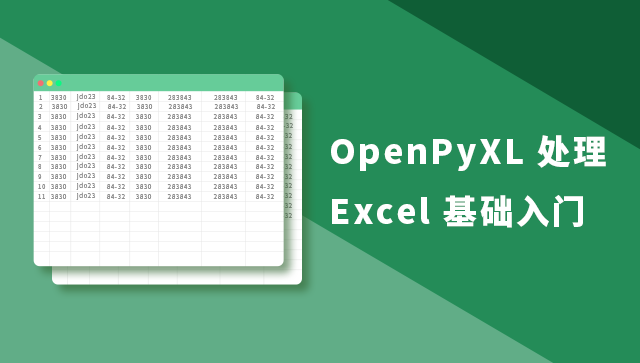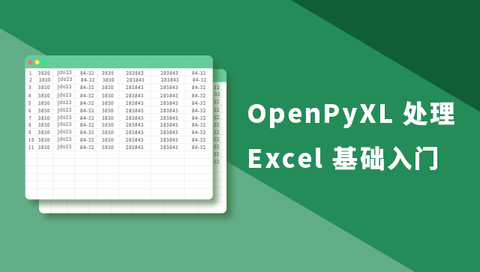Python 究竟有什么法力能让大家如此青睐？

OpenPyXL 处理 Excel 基础入门www.shiyanlou.com• 创建/打开工作簿
• 访问工作表单元及其值
• 保存工作表

### 创建/打开工作簿

``````!wget -nc "https://labfile.oss.aliyuncs.com/courses/1585/shiyanlou.xlsx"
!pip install openpyxl==3.0.3``````

``````from openpyxl import Workbook

wb = Workbook()  # 实例化一个工作簿对象

print(wb)``````

``````from openpyxl import load_workbook

print(wb)``````

`load_workbook` 中可以使用以下几个参数：

• `data_only`：带有公式的单元格是否具有公式（默认具有）或上一次 Excel 读取工作表时存储的值。
• `keep_vba`：设置是否保留任何 Visual Basic 元素（默认保留），可选择保留但是不支持编辑。

``````ws = wb.active  # 获取当前活跃的工作表
print(ws)``````

``````ws = wb.create_sheet() # sheet
ws1 = wb.create_sheet("Mysheet")  # 命名为 Mysheet
ws2 = wb.create_sheet("Mysheet1", 0)  # 新建 Mysheet1 工作表插入到第一个位置
ws3 = wb.create_sheet("Mysheet2", -1)  # 新建 Mysheet2 工作表插入到倒数第二个的位置
ws.title = "shiyanlou"  # 将上述 ws 工作表重命名为 shiyanlou

print("Success")``````

``````ws.sheet_properties.tabColor = "1072BA"  # RGB 格式
print(ws.sheet_properties.tabColor)``````

``````ws = wb["shiyanlou"]
print(ws)``````

``print(wb.sheetnames)``

``````for sheet in wb:
print(sheet.title)``````

``````source = wb.active  # 获取活跃的工作表
target = wb.copy_worksheet(source)  # 上述获取的工作表为其创建副本

print(target)``````

### 访问工作表单元及其值

``````c = ws['A4']  # 将访问 A4 单元格
ws['A4'] = 4  # 对 A4 单元格进行赋值

c.value  # 访问 A4 单元格的值，同 ws['A4'].value``````

``````d = ws.cell(row=4, column=2, value=10)  #  B4 进行赋值

ws['B4'].value  # 访问单元格的值``````

### 访问多个单元格

``````cell_range = ws['A1':'C2']

cell_range``````

``````# 访问列
colC = ws['C']
col_range = ws['C:D']

# 访问行
row10 = ws
row_range = ws[5:10]

print(col_range, row_range)``````

``````for row in ws.iter_rows(min_row=1, max_col=3, max_row=2):
for cell_row in row:
print(cell_row)``````

``````for col in ws.iter_cols(min_row=1, max_col=3, max_row=2):
for cell_col in col:
print(cell_col)``````

``````ws['C9'] = 'hello world'
tuple(ws.rows)``````

``tuple(ws.columns)``

### 访问值

``````for row in ws.values:
for value in row:
print(value)``````

`Worksheet.iter_rows()``Worksheet.iter_cols()` 可以用 `values_only` 参数，只返回单元格的值：

``````for row in ws.iter_rows(min_row=1, max_col=3, max_row=2, values_only=True):
print(row)``````

### 保存工作表

``wb.save("shiyanlou.xlsx")``

OpenPyXL 处理 Excel 基础入门www.shiyanlou.com